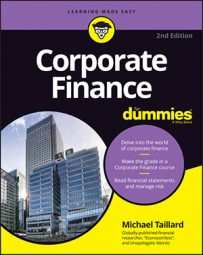##### Corporate Finance For DummiesThe future value of an asset refers to the amount of value that you estimate something will have at any point in the future. Want to know what a machine will be worth after 5 years? Want to know how much your bank account will be worth in 6 months? You can measure both things using future value.

The vast majority of future value calculations are functions of just three things:

• Present value

• Rate

• Time

All future value calculations are just a matter of determining how much revenue an investment will generate over a period of time at the interest rate offered by that particular investment. Two of the most commonly used future value equations in corporate finance involve interest rates. You use the same calculations to determine the cost of debt for a corporation.

## Simple interest

Take a look at this equation:

FV = PV(1 + rt)

The equation shows that for any asset that earns fixed rate interest, the future value (FV) of the asset will be worth the present value (PV) multiplied by the function of interest rate (r) and time duration (t) plus 1.

Here’s a quick example to show you how this equation works: Say you buy an investment for \$100 that yields 1 percent interest per year and hold it for 10 years. To figure out the future value of that investment, simply plug these numbers into the simple interest equation:

FV = \$100(1 + 0.01 x 10)
FV = \$100(1.1)
FV = \$110

When you multiply rate and time in this equation, you get 0.1, and when you multiply that by the PV of \$100, you get \$10. That’s the total amount of increase in nominal value that the interest will have earned over 10 years.

Although that’s good to know, you need to include the total amount you put into the account in the first place (the PV) in order to figure out the future value of the investment as a whole. So you just add 1 to the 0.1, and multiply that by the original \$100, resulting in a future value of \$110.

## Compound interest

Compound interest is similar to simple interest, except that accounts earning compound interest generate interest on the interest earned rather than just on the principal balance. Although this difference adds some complexity to the equation you use to calculate the future value of an investment that earns compound interest, the basic components are still the same, as you can see here:

FV = PV[(1 + r)t]